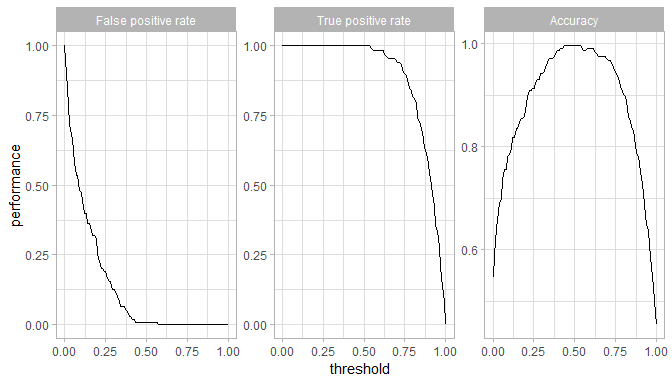# DriveML: Self-Drive machine learning projects

## 1. Introduction

The document introduces the DriveML package and how it can help you to build effortless machine learning binary classification models in short period

DriveML is a series of functions sucha as AutoDataPrep, AutoMAR, autoMLmodel. DriveML automates some of the most difficult machine learning functions such as data exploratory analysis, data pre-processing, feature engineering, model training, model validation, model tuning and model selection.

This package automates following steps on any input dataset for machine learning classification problems

1. Exploratory data analysis using SmartEDA functions
• Generate automated EDA report in HTML format to understand the distributions of the data
2. Data cleaning
• Replacing NA, infinite values
• Removing duplicates
• Cleaning feature names
3. Feature engineering
• Missing at random features
• Missing variable imputation
• Outlier treatment - Oultier flag and imputation with 5th or 95th percentile value
• Date variable transformation
• Bulk interactions for numerical features
• Frequent tranformer for categorical features
• Categorical feature engineering - one hot encoding
• Feature selection using zero variance, correlation and AUC method
4. Model training and validation
• Automated test and validation set creations
• Hyperparameter tuing using random search
• Mutliple binary classification included like logistic regression, randomForest, xgboost, glmnet, rpart
• Model validation using AUC value
• Model plots like training and testing ROC curve, threshold plot
• Probaility scores and model objects
5. Ensemble modelling
• Model ensembling using nnls and nnet method
6. Model Explanation
• Lift plot
• Partial dependence plot
• Feature importance plot
7. Model report
• model output in rmarkdown html format

To summarize, DriveML package helps in getting the complete Machine learning classification model just by running the function instead of writing lengthy r code.

This package performs ML models in R using MLR package

#### Missing not at random features

Algorithm: Missing at random features

1. Select all the missing features X_i where i=1,2,…..,N.
2. For i=1 to N:
• Define Y_i, which will have value of 1 if X_i has a missing value, 0 if X_i is not having missing value
• Impute all X_(i+1 ) 〖to X〗_(N ) variables using imputation method
• Fit binary classifier f_m to the training data using Y_i ~ X_(i+1 ) 〖+⋯+ X〗_(N )
• Calculate AUC ∝_ivalue between actual Y_i and predicted Y ̂_i
• If ∝_i is low then the missing values in X_i are missing at random,Y_i to be dropped
• Repeat steps 1 to 4 for all the independent variables in the original dataset

## 2. Functionalities of DriveML

The DriveML R package has four unique functionalities as

1. Data Exploration
• SmartEDA has a complete exploratory data analysis function
2. Data preparations
• autoDataPrep function to generate a novel features based on the functional understanding of the dataset
3. Machine learning models
• autoMLmodel function to develope baseline machine learning models using regression and tree based classfication techniques
4. Model report
• autoMLReport function to print the machine learning model outcome in HTML format

## 3. DriveML: Machine learning classfication model

This database contains 76 attributes, but all published experiments refer to using a subset of 14 of them. In particular, the Cleveland database is the only one that has been used by ML researchers to this date. The “goal” field refers to the presence of heart disease in the patient. It is integer valued from 0 (no presence) to 4.

Data Source Kaggle.

Install the package “DriveML” to get the example data set.

install.packages("DriveML")
library("DriveML")
library("SmartEDA")
## Load sample dataset from ISLR pacakge
heart = DriveML::heart

more detailed attribute information is there in DriveML help page

### 3.1 Data Exploration

For data exploratory analysis used SmartEDA package

#### Overview of the data

Understanding the dimensions of the dataset, variable names, overall missing summary and data types of each variables

# Overview of the data - Type = 1
ExpData(data=heart,type=1)

# Structure of the data - Type = 2
ExpData(data=heart,type=2)
• Overview of the data
Descriptions Obs
Sample size (Nrow) 303
No. of Variables (Ncol) 14
No. of Numeric Variables 14
No. of Factor Variables 0
No. of Text Variables 0
No. of Logical Variables 0
No. of Unique Variables 0
No. of Date Variables 0
No. of Zero variance Variables (Uniform) 0
%. of Variables having complete cases 100% (14)
%. of Variables having <50% missing cases 0% (0)
%. of Variables having >50% missing cases 0% (0)
%. of Variables having >90% missing cases 0% (0)
• Structure of the data
S.no Variable Name Variable Type % of Missing No. of Unique values
1 age integer 0 41
2 sex integer 0 2
3 cp integer 0 4
4 trestbps integer 0 49
5 chol integer 0 152
6 fbs integer 0 2
7 restecg integer 0 3
8 thalach integer 0 91
9 exang integer 0 2
10 oldpeak numeric 0 40
11 slope integer 0 3
12 ca integer 0 5
13 thal integer 0 4
14 target_var integer 0 2

#### Summary of numerical variables

ExpNumStat(heart,by="GA",gp="target_var",Qnt=seq(0,1,0.1),MesofShape=2,Outlier=TRUE,round=2)

#### Distributions of Numerical variables

• Box plots for all numerical variables vs categorical dependent variable - Bivariate comparision only with categories

Boxplot for all the numeric attributes by each category of target_var

plot4 <- ExpNumViz(heart,target="target_var",type=1,nlim=3,fname=NULL,Page=c(2,2),sample=8)
plot4[]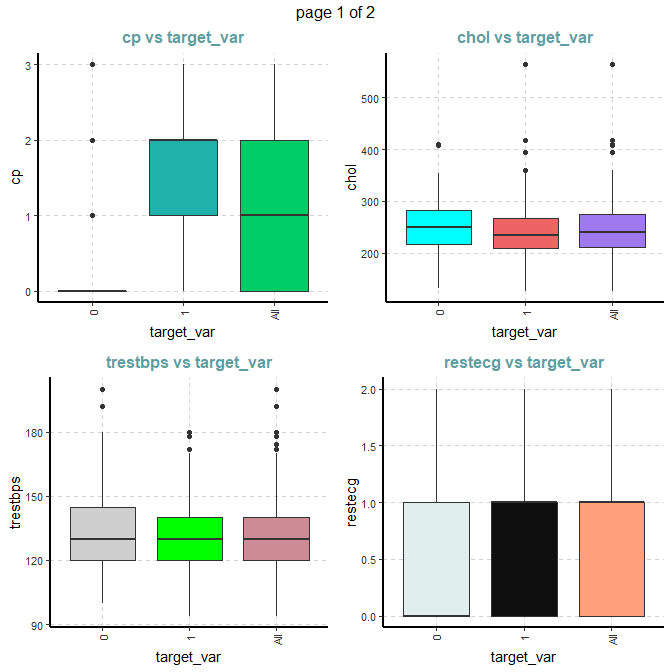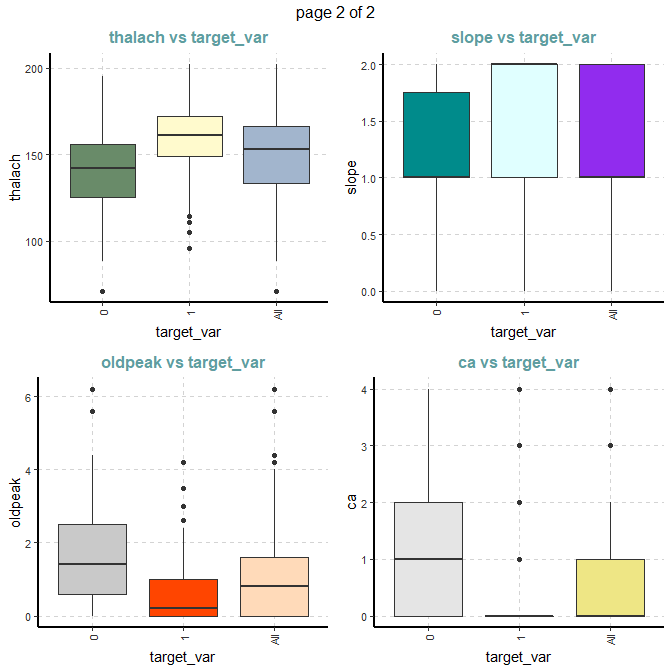#### Summary of categorical variables

Cross tabulation with target_var variable

• Custom tables between all categorical independent variables and target_var variable target_var
ExpCTable(Carseats,Target="Urban",margin=1,clim=10,nlim=3,round=2,bin=NULL,per=F)
VARIABLE CATEGORY target_var:0 target_var:1 TOTAL
sex 0 24 72 96
sex 1 114 93 207
sex TOTAL 138 165 303
fbs 0 116 142 258
fbs 1 22 23 45
fbs TOTAL 138 165 303
restecg 0 79 68 147
restecg 1 56 96 152
restecg 2 3 1 4
restecg TOTAL 138 165 303
exang 0 62 142 204
exang 1 76 23 99
exang TOTAL 138 165 303
slope 0 12 9 21
slope 1 91 49 140
slope 2 35 107 142
slope TOTAL 138 165 303
target_var 0 138 0 138
target_var 1 0 165 165
target_var TOTAL 138 165 303

#### Distributions of categorical variables

Stacked bar plot with vertical or horizontal bars for all categorical variables

plot5 <- ExpCatViz(heart,target = "target_var", fname = NULL, clim=5,col=c("slateblue4","slateblue1"),margin=2,Page = c(2,1),sample=2)
plot5[]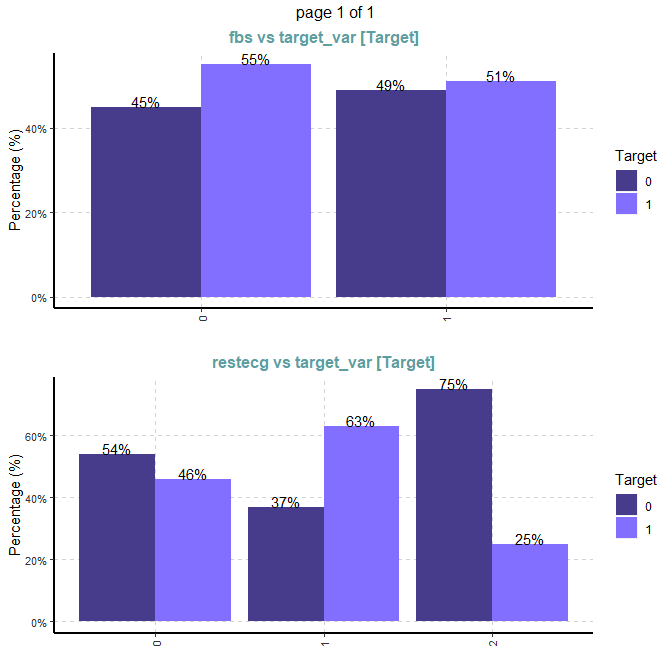#### Outlier analysis using boxplot

ExpOutliers(heart, varlist = c("oldpeak","trestbps","chol"), method = "boxplot",  treatment = "mean", capping = c(0.1, 0.9))
Category oldpeak trestbps chol
Lower cap : 0.1 0 110 188
Upper cap : 0.9 2.8 152 308.8
Lower bound -2.4 90 115.75
Upper bound 4 170 369.75
Num of outliers 5 9 5
Lower outlier case
Upper outlier case 102,205,222,251,292 9,102,111,204,224,242,249,261,267 29,86,97,221,247
Mean before 1.04 131.62 246.26
Mean after 0.97 130.1 243.04
Median before 0.8 130 240
Median after 0.65 130 240

### 3.2 Data preparations using autoDataprep

dateprep <- autoDataprep(data = heart,
target = 'target_var',
missimpute = 'default',
auto_mar = FALSE,
mar_object = NULL,
dummyvar = TRUE,
char_var_limit = 15,
aucv = 0.002,
corr = 0.98,
outlier_flag = TRUE,
uid = NULL,
onlykeep = NULL,
drop = NULL)
printautoDataprep(dateprep)
## Data preparation result
## Call:
##  autoDataprep(data = heart, target = "target_var", missimpute = "default",      auto_mar = FALSE, mar_object = NULL, dummyvar = TRUE, char_var_limit = 15,      aucv = 0.002, corr = 0.98, outlier_flag = TRUE, uid = NULL,      onlykeep = NULL, drop = NULL)
##
##  *** Data preparation summary ***
## Total no. of columns available in the data set:  14
## No. of numeric columns:                          8
## No. of factor / character columns:               0
## No. of date columns:                             0
## No. of logical columns:                          0
## No. of unique columns:                           0
## No. of MAR columns:                              0
## No. of dummy variables created:                  0
##
##  *** Variable reduction ***
## Step 1 - Checked and removed useless variables:         6
## Step 2 - No. of variables before fetature reduction:    22
## Step 3 - No. of zero variance columns (Constant):       0
## Step 4 - No. of high correlated or bijection columns:   3
## Step 5 - No. of low AUC valued columns:                 2
## *Final number of columns considered for ML model:       17
##
##  *** Data preparation highlights ***
## Missing replaced with {
## --> factor = imputeMode()
## --> integer = imputeMean()
## --> numeric = imputeMedian()
## --> character = imputeMode() }
train_data <- dateprep$master_data ### 3.3 Machine learning models using autoMLmodel Automated training, tuning and validation of machine learning models. This function includes following binary classification techniques • Logistic regression - logreg • Regularised regression - glmnet • Extreme gradient boosting - xgboost • Random forest - randomForest • Random forest - ranger • Decision tree - rpart mymodel <- autoMLmodel( train = heart, test = NULL, target = 'target_var', testSplit = 0.2, tuneIters = 10, tuneType = "random", models = "all", varImp = 10, liftGroup = 50, maxObs = 4000, uid = NULL, htmlreport = FALSE, seed = 1991) ### 3.3 Model output Model performance Model Fitting time Scoring time Train AUC Test AUC Accuracy Precision Recall F1_score glmnet 2.558 secs 0.008 secs 0.928 0.908 0.820 0.824 0.848 0.836 logreg 2.513 secs 0.004 secs 0.929 0.906 0.820 0.824 0.848 0.836 randomForest 2.785 secs 0.012 secs 1.000 0.877 0.754 0.765 0.788 0.776 ranger 2.981 secs 0.044 secs 0.999 0.900 0.803 0.784 0.879 0.829 xgboost 2.938 secs 0.004 secs 0.996 0.907 0.820 0.806 0.879 0.841 rpart 2.559 secs 0.004 secs 0.927 0.814 0.738 0.730 0.818 0.771 Randomforest model ROC curve and variable importance Training data set ROC TrainROC <- mymodel$trainedModels$randomForest$modelPlots$TrainROC TrainROC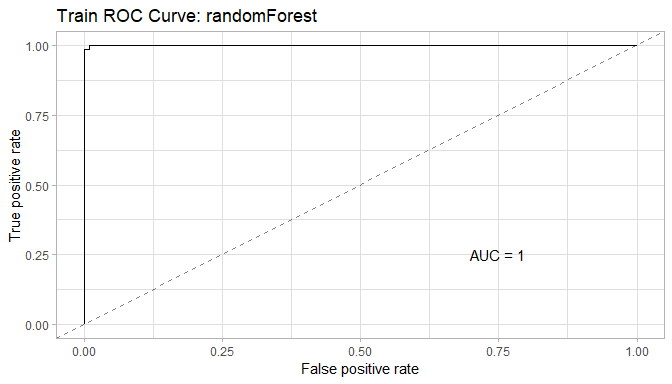Test data set ROC TestROC <- mymodel$trainedModels$randomForest$modelPlots$TestROC TestROC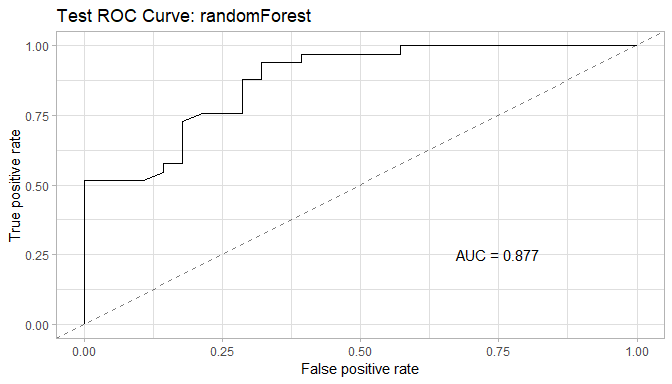Variable importance VarImp <- mymodel$trainedModels$randomForest$modelPlots$VarImp VarImp ## []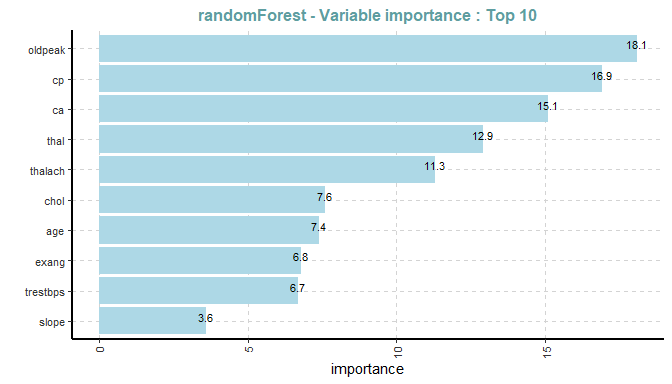Threshold Threshold <- mymodel$trainedModels$randomForest$modelPlots\$Threshold
Threshold